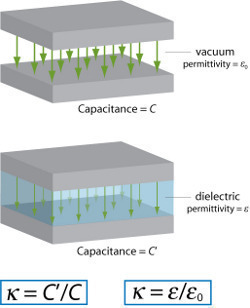•• # Dielectric Constant

A dielectric constant measures the extent that a material concentrates electrostatic flux and is also known as the relative static permittivity, static dielectric constant, and relative dielectric constant. It is essential when determining if a substance can be used in a capacitor or various chemistry and physics applications. A material’s dielectric constant value must be known before using it to make optical fibers or coaxial cables. Although the term is commonly used, it may have different mathematical connotations depending on the context of its application. The dielectric constant refers to either the static or frequency-dependent relative permittivity.

## How to Calculate the Dielectric Constant

Step 1 – Determine the material’s dielectric constant, which is the ratio of test material static permittivity to vacuum static permittivity. The formula is er = es/e0, where er stands for the dielectric constant, es stands for the static permittivity of the material being tested, and e0 is the vacuum static permittivity, which has a value of 1 by definition.Step 2 – Note the dielectric constant’s units, which is a number representing the ratio of es and e0.

Step 3 – Measure the capacitor’s capacitance with test material placed between the plates and assign this value to Cx. Repeat this process with a vacuum between the plates instead and assign the value to C0.

Step 4 – Divide Cx by Co in order to determine the dielectric constant. If the electromagnetic field changes over time, the value is determined based on the frequency of change and is more commonly referred to as relative permittivity.

Step 5 – The following formula determines the dielectric constant from the static electric susceptibility: er = 1 +Xe. Xe is the static electricity of the susceptibility of the material being tested.

264 queries in 0.467 seconds.